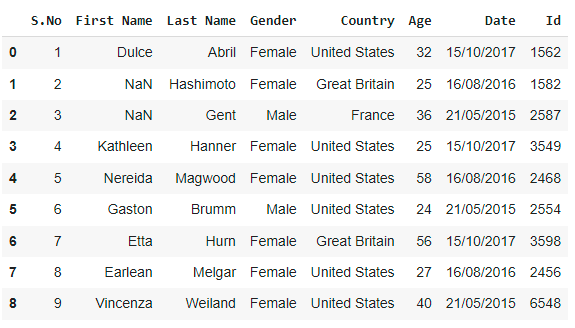# How to Import an Excel File into Python using Pandas?

The dataset may not always be available in CSV format. As a result, Pandas includes functions for converting datasets in different formats to Data frames. The format of an excel file is ‘.xlsx’.

Before we begin, we must first install a few libraries as shown below:

```pip install pandas
pip install xlrd```

We must use the pandas.read_excel() function to import an Excel file into Python using Pandas.

Syntax:

`pandas.read_excel(io, sheet_name=0, header=0, names=None,….)`

Return Value: It returns a Dataframe or a dictionary of Dataframes.

Let us take an example of demo.xlsx excel spreadsheet as shown below: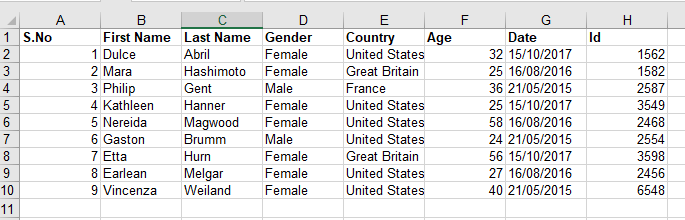## How to Import an Excel File into Python using Pandas?

Approach:

• Import pandas module as pd using the import keyword.
• Read the excel file using the read_excel() function and store it as a DataFrame.
• The Exit of the Program.

Below is the implementation:

```# Import pandas module as pd using the import keyword
import pandas as pd

# store it as a DataFrame
data_frme```

Output: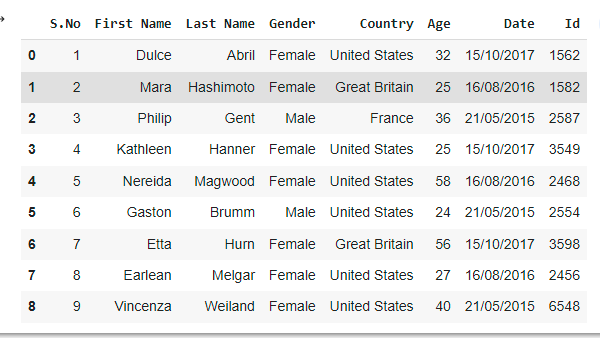Example2: Selecting a specific column

Approach:

• Import pandas module as pd using the import keyword.
• Select a specific column of the excel file using the index_col by passing the column index as an argument to it.
• The Exit of the Program.
```# Import pandas module as pd using the import keyword
import pandas as pd

# Select a specific column of the excel file using the index_col by passing the
# column index as an argument to it
index_col = 0)
data_frme```

Output: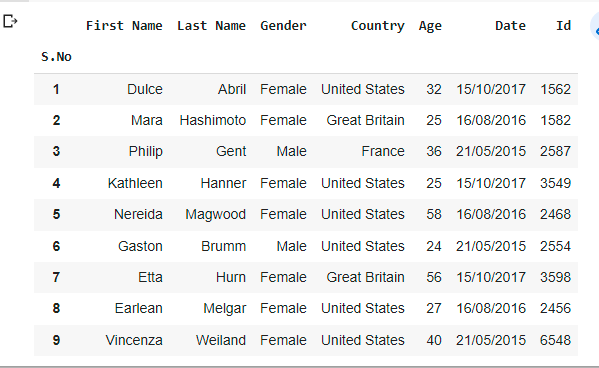Example3:

If we don’t want the initial heading of the columns, you can change it to indexes by using the “header” argument.

```# Import pandas module as pd using the import keyword
import pandas as pd

# Read the excel file using the read_excel() function by passing the
# file name and header= None as the arguments to it.
# (It modifies the header with indexes)
# store it as a DataFrame
data_frme```

Output: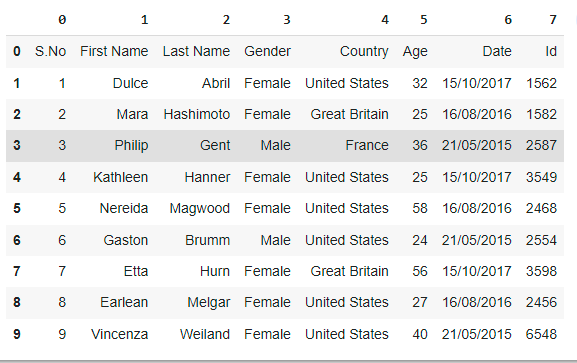Example4: Changing the datatype of columns

If we wish to modify or change the data type of a certain column, use the “dtype” argument.

```# Import pandas module as pd using the import keyword
import pandas as pd

# Change the datatype of coulums(Gender, Age) using the dtype parameter
# and store it in a variable
dtype = {"Gender": str,
"Age":float})
data_frme```

Output: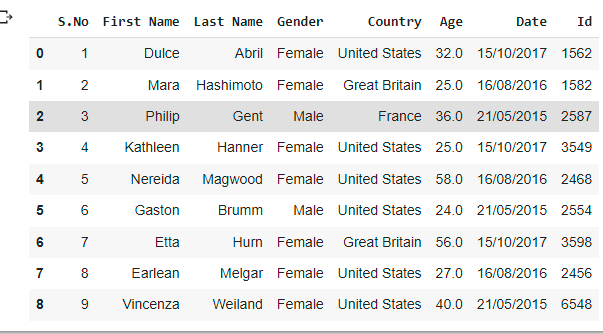Example5:

If we have unknown values, you can handle them with the “na_values” argument. It will convert the previously specified unknown values to “NaN.”

```# Import pandas module as pd using the import keyword
import pandas as pd

# Convert the unknown values to NaN using the na_values argument